# HC Verma Solutions Class 12 Chapter 5 Specific Heat Capacities Of Gases

HC Verma Solutions Class 12 Chapter 5 Specific Heat Capacities of Gases are an in-depth guide to solve problems given in the chapter. The solutions contain detailed answers that will help students develop better analytical skills as well as get better insights into the concepts. This will aid in their exam preparation and they will be better prepare for the exams including board and competitive ones like JEE.

The HC Verma Solutions have been created by subject experts and students who use the solutions will improve their problem-solving approach to complex questions on Specific Heat Capacities of gases. Students of 12th grade can make use of this material to study smartly and achieve better performance in the exams.

Students can access the HC Verma Vol 2 solution PDF from the link provided below.

## Topics Related To Specific Heat Capacities Of Gases

As for the chapter, it covers various topics related to specific heat capacities of gases. Some of the important ones include;

• Types of Specific Heat Of Gases
• Equipartition Of Energy
• Relation and Determination

## Class 12 Important Questions In Chapter 5

1. Will a gas have one specific heat capacity or more than two? Can specific heat capacities of gas be measured?

2. Is it possible to define specific heat capacity at constant temperature and for an adiabatic process?

3. Can the isothermal process or adiabatic process be used to connect two states of an ideal gas?

4. The pressure on ideal gas is increased by 0’5 per cent in an isothermal process. By what per cent will the volume decrease?

(a) 0-7% a) 0-25% (c) 0-5% (d) 1%

5. A gas is kept in a container of finite conductivity. However, the gas is suddenly compressed. What is the process involved?

(a) nearly adiabatic (b) nearly isothermal (c) may be very nearly adiabatic (d) may be very nearly isothermal.

## HC Verma Solutions Vol 2 Specific Heat Capacities of gases Chapter 5

Question 1: A vessel containing one mole of a monatomic ideal gas (molecular weight = 20 g mol–1) is moving on a floor at a speed of 50 ms–1. The vessel is stopped suddenly. Assuming that the mechanical energy lost has gone into the internal energy of the gas, find the rise in its temperature.

Solution:

Change in internal energy = ΔU = nCv ΔT …(1)

Given: n = 1, Cv = 3R/2 = 12.471 J/mol/K and ΔT = rise in temperature.

and Mechanical energy lost = E = -m [(vi2-vf2)/2] ….(2)

Equating, (1) and (2) and substituting the values, we have

=> [0.02 x (2500-0)]/2 = 12.471 x ΔT

=> ΔT = 2 K

Question 2: 5g of a gas is contained in a rigid container and is heated from 15°C to 25°C. Specific heat capacity of the gas at constant volume is 0.172 cal g–1°C–1 and the mechanical equivalent of heat is 4.2 J cal–1. Calculate the change in the internal energy of the gas.

Solution:

we know, Heat(Joule) = Mechanical equivalent of heat × Heat(cal)

dQ = du + dW

For rigid body, V = 0

So dW = 0

=> dQ = du

and Q = ms dt = 5×0.172×10 = 8.6 cal = 8.6×4.2 = 36.12 Joule

Question 3: The figure shows a cylindrical container containing oxygen (γ = 1.4) and closed by a 50 kg frictionless piston. The area of cross section is 100 cm2, atmospheric pressure is 100 kPa and g is 10 ms–2. The cylinder is slowly heated for some time. Find the amount of heat supplied to the gas if the piston moves out through a distance of 20 cm.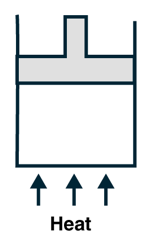Solution:

Mass of piston(m) = 50 kg

Area(A) = 100 cm2 = (100×10-4)m2

And γ = 1.4

Atmospheric pressure = 100 kPa = 1,00,000 Pa

Pressure exerted by piston = force/area = mg/area

= (50×10)/(100×10-4) Pa = 50,000 Pa

Total pressure(P) = (50,000 + 1,00,000)Pa = 1,50,000 Pa

Work done = Pressure x change in volume = P dV

dV = distance moved by piston x Area = 20×100 = 2,000 cm3 = 2,000 x 10-6 m3 = 2 x 10-3 m3

So, Work done = (1,50,000 x 2 x 10-3) J = 300 J

We know, Work done, W = P∆V = n R dT

=> dT = 300/nR

Now,

dQ = nCP dt = 300 x CP/R

Also given, γ = 1.4 = CP/CV and CP + CV = R

=> CP = 7R/2, CV = 5R/2.

=> dQ = 300/R x 7R/2 = 1050 J

Question 4: The specific heat capacities of hydrogen at constant volume and at constant pressure are 2.4 cal g−1 °C−1 and 3.4 cal g−1 °C−1 respectively. The molecular weight of hydrogen is 2 g mol−1 and the gas constant, R = 8.3 × 107 erg °C−1mol−1. Calculate the value of J

Solution:

The gas constant = R= (m X CP ) – (m X CV) = 2 × (CP – CV)= 2×J

Given: CV =2.4 cal g-1 oC-1 and CP = 3.4 cal g-1 oC-1

Molecular mass of hydrogen = 2 g mol-1

Gas constant = R = 8.3 × 107 g mol

Now, 2J = R

=> 2J = 8.3 × 107 erg/ mol oC

or J = 4.15 × 10^7 erg/cal

Question 5: The ratio of the molar heat capacities of an ideal gas is Cp/Cv = 7/6. Calculate the change in internal energy of 1.0 mole of the gas when its temperature is raised by 50 K

(a) keeping the pressure constant,

(b) keeping the volume constant and

Solution:

Cp/Cv = 7/6 => Cv = 6Cp/7

n = number of moles = 1

dT = change in temperature = 50K

(a) change in internal energy = dU = nCvdT

Also, cp – cv = R

=> Cp – 6Cp/7 = Cp/7 = R

=> Cp = 7R

Therefore, Cv = Cp – R = 6R = (6 X 8.314)J/mol/K

=> dU = 1 x (6 X 8.314) x 50 = 2494.2 J

(b) Volume constant, so dV = 0

By First law of thermodynamics: dU = dQ – dW

Also, dW = P dV = 0

As, dQ = nCv dT

=> dU = dQ = nCv dT = 1 x (6 X 8.314) x 50 = 2494.2 J

(c) Adiabatic process: dQ(heat change)= 0

We know, dQ = dU + dW.

=> dU = -dW

Where dU = change in internal energy.

Also, For an adiabatic process, dW = dT/(𝛾-1).

and 𝛾 = Cp/Cv = 7/6 (Given)

=> dU = -dW – (-nR dT)/(𝛾-1) = 2494.2 J

Question 6: A sample of air weighing 1.18 g occupies 1.0 × 103 cm3 when kept at 300K and 1.0 × 105 Pa. When 2.0 cal of heat is added to it at constant volume, its temperature increases by 1°C. Calculate the amount of heat needed to increase the temperature of air by 1°C at constant pressure if the mechanical equivalent of heat is 4.2 × 107 erg cal-1. Assume that air behaves as an ideal gas.

Solution:

Ideal gas equation: PV = nRT

=> n = PV/RT = [100000×0.001]/[8.314 x300] = 0.04 mol

By First law of thermodynamics: dQ = dU + dW = dU + PdV

=> dW = PdV

Since volume is constant, dV = 0 => dW = 0.

Hence, dQ = dU.

dQ = Heat = 2 cal (Given) and dQ = n CvdT

=> 2 = 0.04 mol x Cv x 1K

=> Cv = 50 cal/mol/K = (50 x 4.2 × 107)erg/cal x cal/mol/K = 2.1 x 109 erg/mol/K = 210 J/mol/K

We know, Cp = (Cv + R)

=> Cp = (210+8.314) J/mol/K = 218.314 J/mol/K

Therefore, heat required to raise the temperature by 1°C at constant pressure = nCpdT

=> Heat = (0.04 x 218.314 x 1) J = 8.737 J = (8.737/4.2) cal = 2.08 cal (since 1 J = 4.2 cal)

Question 7: An ideal gas expands from 100 cm3 to 200 cm3 at a constant pressure of 2.0 × 105 Pa when 50J of heat is supplied to it. Calculate

(a) the change in internal energy of the gas.

(b) the number of moles in the gas if the initial temperature is 300K.

(c) the molar heat capacity CP at constant pressure and

(d) the molar heat capacity CV at constant volume.

Solution:

(a) dU = dQ – PdV = (50 – (2.0 × 105 x 10-4)) J = 30 J

(b) For constant pressure, from equation of state PV/T = constant

=> V1/T1 = V2/T2

Given: V1 = 100 cm3, V2 = 200 cm3, T1 = 300K

=> T2 = 600 K

So, dT = T2 – T1 = 300 K

Therefore, PdV = nRdT

=> 2.0 × 105 x 10-4 = n x 8.314 x 300

=> n = 0.008 mol

(c) At constant pressure

dQ = 50 = nCpdT

=> 50 = 0.008 x Cp x 300

=> Cp = 20.83 J/mol/K

(d) At constant volume, dU = dQ = nCvdT

dU = 30J from part (a)

=> 30 = 0.008 x Cv x 300

=> Cv = 12.5 J/mol/K.

Question 8: An amount Q of heat is added to a monatomic ideal gas in a process in which the gas performs a work Q/2 on its surrounding. Find the molar heat capacity for the process.

Solution:

dQ = W + dU

For monoatomic gas: dU = Q – Q/2 = Q/2

and Cv = (3R/2) J/kg/mol

We know, dU = nCv dT and dQ = nCdT

When dU = dQ

=> nCv dT = nC dT/2

=> c = 2 Cv

=> C = 2*(3R/2) = 3R J/kg/mol.

Question 9: An ideal gas is taken through a process in which the pressure and the volume are changed according to the equation p = kV. Show that the molar heat capacity of the gas for the process is given by C = Cv + R/2.

Solution:

P = kV … (1) (Given)

Multiplying by dV on both sides, we get

PdV = kVdV

on Integrating from V = V1 to V2, we get

∫dV = k/2 [V22 – V12]

Equation of state of ideal gas: PV = nRT = constant … (2)

We can write, V1 = nRT1/P1

P1, V1, T1 = Pressure, volume, temperature of first gas

Since P1 = kV1

kV12 = nRT1. Similarly, KV22 = nRT2, where P2, V2, T2 = Pressure, volume, temperature of second gas

on substituting, the above equation becomes,

=> (k/2) x nR/K [T2 – T1] = nR/2 dT …(3)

Now, V = nRT/P

=> V2 = nRT/k …(4)

[As, P = kV ]

Using, first law of thermodynamics

Q = U + ഽPdV

=> nCdT = nCv dT + (nR/2)dT

(As, Q = nCdT and U = nCv dT)

=> C = Cv + nR/2 . Hence proved.

Question 10: An ideal gas (CP/CV = γ) is taken through a process in which the pressure and the volume vary as p = aVb. Find the value of b for which the specific heat capacity in the process is zero.

Solution:

Given: p = aVb …(1)

specific heat capacity is zero …(given)

Therefore, process is essentially an adiabatic process.

So, PVγ = Constant

=> P = c/Vγ = c v …(2)

Comparing (1) and (2), we get

a = c and b = -γ

Question 11: Two ideal gases have the same value of CP/CV = γ. What will be the value of this ratio for a mixture of the two gases in the ratio 1: 2?

Solution:

Cp/Cv = γ (Given)

We know, R= Cp – Cv

Now, Cv = R/(γ-1)

For the first ideal gas:

Cv1 = R/(γ-1) and Cp1 = Rγ/(γ-1)

Where Cp1 and CV1 is the molar heat capacity at constant pressure and constant volume.

For the second ideal gas:

Cv2 = R/(γ-1) and Cp2 = Rγ/(γ-1)

Where C p2 and CV2 is the molar heat capacity at constant pressure and constant volume

Since we are given, n1 = n2 = 1:2

dU1 = nCV1 dT and dU2 = 2n CV2 dT

When gas is mixed: nCV1 dT + 2n CV2 dT = 3 n CV dT

=> CV = (1/3) [Cv1 + 2Cv2]

on substituting C v1 and Cv2, we get

Cp/Cv = γ

Question 12: A mixture contains 1 mole of helium (CP = 2.5 R, CV = 1.5R) and 1 mole of hydrogen (CP = 3.5 R, CV = 2.5 R). Calculate the values of CP, CV and γ for the mixture.

Solution:

CP1 = 2.5 R, CV1= 1.5R for helium

CP2 = 3.5 R, CV2 = 2.5 R for hydrogen

n1=n2=1

We know, dU = nCv dT

For the mixture,

(n1 + n2)Cv dT = n1Cv1 dT + n2Cv2 dT

On substituting the values,

Cv = 2R

Also, CP = Cv + R = 3R

=> γ = Cp/Cv = 1.5

Question 13: Half mole of an ideal gas (γ = 5/3) is taken through the cycle abcda as shown in figure. Take R = (25/3) J/Kmol.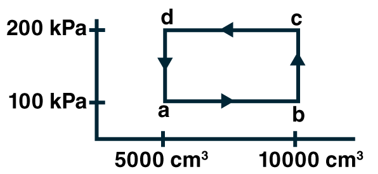(a) Find the temperature of the gas in the states a, b, c and d.

(b) Find the amount of heat supplied in the processes ab and bc.

(c) Find the amount of heat liberated in the processes cd and da.

Solution:

Given, n = 1/2, γ = 5/3, R = 25/3 J/Kmol

a) By ideal gas equation, PV = nRT

Here, temperature at a, Ta = PV/nR

= [5000×10-6x100x103]/[(1/2 x (25/3)]

= 120 K

Here, temperature at b

Tb = PV/nR = [10000×10-6x100x103]/[(1/2 x (25/3)]

= 240K

Here, temperature at c

Tc = PV/nR = [1000×10-6x200x103]/[(1/2 x (25/3)]

= 480K

Here, temperature at d

Td = PV/nR = [5000×10-6x200x103]/[(1/2 x (25/3)]

= 240K

(b) For ab process

dQ = ncp dT = (1/2) x R/(γ -1) [Tc – Tb] = 1500 J

(c) Heat liberated in cd

dQ = -ncp dT = (-1/2) x R/(γ -1) [Td – Tc] = 2500 J

Heat liberated in da:

dQ = -ncv dT = (1/2) x R/(γ -1) [Ta – Td] = 750 J

Question 14: An ideal gas (γ = 1.67) is taken through the process abc shown in figure. The temperature at the point a is 300K. Calculate

(a) the temperature at b and c,

(b) the work done in the process,

(c) the amount of heat supplied in the path ab and in the path bc and

(d) the change in the internal energy of the gas in the process.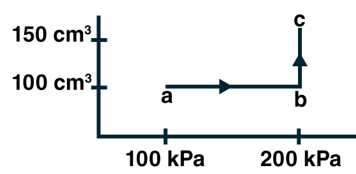Solution:

(a) For the process ab volume is constant

Ideal gas equation, P1/T1 = P2/T2

=> 100/300 = 200/T2

=> T2 = 600 k

For the process bc, pressure is constant.

By ideal gas equation, V2/T2 = V3/T3

=> 100/600 = 150/T3

=> T3 = 900 k

(b) Process ab is isochoric i.e. Wab = 0

For process bc, P=200 kPa, change in volume is 50 cm3 from b to c

Now, work done = P dV = 200×103x50x10-6 = 10 J

(c) first law of thermodynamics: dQ = dU + dW

As ab is isochoric process dW=0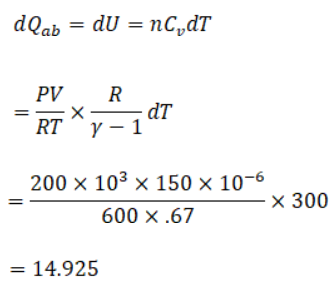Again,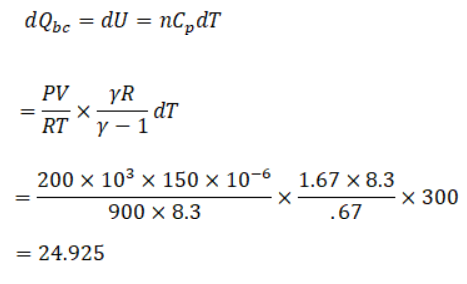(d) dQ = dU + W

or dU = dQ – W

= (24.925+14.925) – 10

= 29.85

Question 15: In Joly’s differential steam calorimeter, 3g of an ideal gas is contained in a rigid closed sphere at 20°C. The sphere is heated by steam at 100°C and it is found that an extra 0.095 g of steam has condensed into water as the temperature of the gas becomes constant. Calculate the specific heat capacity of the gas in J g–1 K–1. The latent heat of vaporization of water = 540 cal g–1.

Solution:

In Joly’s differential steam calorimeter,

Cv = 2mL/[m12 – θ1]

Given:

m1 = Mass of gas present = 3 g, θ1 = 20°C, θ2 = 100°C

m2 = Mass of steam condensed = 0.095 g, L = 540 Cal/g = 540 × 4.2 J/g

=> Cv = [0.095x540x4.2]/[3×80]

= 0.89 J/gK

Question 16: The volume of an ideal gas (γ = 1.5) is changed adiabatically from 4.00 litres to 3.00 litres. Find the ratio of

(a) the final pressure to the initial pressure and

(b) the final temperature to the initial temperature.

Solution:

(a)Since it is an adiabatic process, So PVγ = Constant

P2/P1 = (V1/V2) γ = 1.54

(b) In this case, TVγ-1 = Constant

T1V1(γ -1) = T2V2(γ -1)

=> T2/T1 = (V1/V2) (γ -1) = (4/3)(1.5-1) = 1.154

Question 17: An ideal gas at pressure 2.5 × 105 Pa and temperature 300K occupies 100 cc. It is adiabatically compressed to half its original volume. Calculate

(a) the final pressure,

(b) the final temperature and

(c) the work done by the gas in the process. Take γ = 1.5.

Solution:

Given, P1 = 2.5 × 105 Pa, V1 = 100 cc, T1 = 300 K, V2 = 50 cc and γ = 1.5

(a)Since it is an adiabatic process, So PVγ = Constant

P2/P1 = (V1/V2)γ

Substituting values, we get

P2 = 7.1 x 105 Pa

(b) In this case, TV(γ -1) = Constant

T1V1(γ -1) = T2V2(γ -1)

=> T2/T1 = (V1/V2)(γ-1)

Substituting values, we get

T2 = 300 x 20.5 = 425 K

Question 18: Air (γ = 1.4) is pumped at 2 atm pressure in a motor tyre at 20°C. If the tyre suddenly bursts, what would be the temperature of the air coming out of the tyre. Neglect any mixing with the atmospheric air.

Solution:

Given γ = 1.4, P1 = 2 atm, P2 = 1 atm, T1 = 20°C = 293 K

Here bursting of tire is an adiabatic process,

T1γ P1(1-γ ) = T2γ P2(1-γ )

=> T2γ = [T1γ P1(1-γ ) ]/ P2(1-γ )

=> T21.4 = 2153.78

=> T2 = (2153.78)1/4 = 240.3 K

Question 19: A gas is enclosed in a cylindrical can fitted with a piston. The walls of the can and the piston are adiabatic. The initial pressure, volume and temperature of the gas are 100 kPa, 400 cm3 and 300 K respectively. The ratio of the specific heat capacities of the gas is CP/CV = 1.5. Find the pressure and the temperature of the gas if it is

(a) suddenly compressed

(b) slowly compressed to 100 cm3.

Solution:

Given, V1 = 400 cm3 ; P1 = 100 KPa = 105 Pa ; T1 = 300 K,

and CP/CV = 1.5

(a) Suddenly compressed to V2 = 100 cm3 [adiabatic process]

=> PVγ = const.

P2/P1 = (V1/V2)γ = (400/100)1.5

=> P2 = 105 x 41.5 = 800 KPa

Also, T1 P1 1-γ = T2P2 1-γ

=> T2 = [T1 P1 1-γ ]/P2 1-γ = 300 x (100/400)-0.5 = 600 K

(b) Even though the container is slowly compressed the walls are adiabatic so heat transferred is zero. Thus the values remain unchanged.

=> P2 = 800 KPa and T2 = 600 K

Question 20: The initial pressure and volume of a given mass of a gas (CP/CV = γ) are P0 and V0. The gas can exchange heat with the surrounding.

(a) It is slowly compressed to a volume V0/2 and then suddenly compressed to V0/4. Find the final pressure.

(b) If the gas is suddenly compressed form the volume V0 to V0/2 and then slowly compressed to V0/4, what will be the final pressure?

Solution:

Here, CP/CV = γ ; P0 = Initial Pressure and V0 = Initial Volume

(a)

(i) Isothermal compression, P1V1 = P2V2

=> P0V0 = P2V0/2

=> P2 = 2 P0

(ii) Adiabatic compression, PVγ = Constant

Now, P3/P2 = (V2/V3) γ

Put V2 = V0/2 and V3 = V0/4 and P2 = 2P0

=> P3 = P0 (2)(γ+1)

(b) Since the volume is suddenly compressed.

Let P2 and V2 = V0/2 be the pressure and volume after compression

P2/P1 = (V1/V2)γ = [v0/(V0/2)]γ = (2)γ

Since P1 = P0

=> P2 = P0 (2)^γ

As, volume is slowly compressed, temperature remains constant [Isothermal compression]

P2 V2 = P3 V3

=> P0 x 2γ (V0/2) = P3 x (V0/4)

=> P3 = P0 2γ+1

Question 21: Consider a given sample of an ideal gas (CP/CV = γ) having initial pressure P0 and volume V0.

(a) The gas is isothermally taken to a pressure P0/2 and from there adiabatically to a pressure P0/4. Find the final volume.

(b) The gas is brought back to its initial state. It is adiabatically taken to a pressure P0/2 and from there isothermally to a pressure P0/4. Find the final volume.

Solution:

Here, CP/CV = γ ; P0 = Initial Pressure and V0 = Initial Volume

(a)

(i) Isothermal compression, P1V1 = P2V2

Here P2 = P0/2

=> P0 V0 = (P0/2) V2

=> V2 = 2 V0

(ii) Adiabatic compression, PVγ = Constant

Here P3 = P0/4

Now, P3/P2 = (V2/V3)γ

Put V2 = P0/4 and V3 = P0/2 and V2 = 2V0

=> V3 = V0 (2)(1/γ + 1)

Here P1 = P0, P2 = P0/2

Let V1 = V0 be the initial volume and V2 be the volume after process.

and PVγ = Constant

Now,

P2/P1 = (V1/V2)γ = [(P0/2)/P0]γ = (V0/V2) γ

=> V2 = V0 (2)1/γ

Again,

After isothermal process,

Let P3 = P0/4 and V3 be the pressure and volume

=> P2 V2 = P3V3

=> (P0/2) x V0 x 21/γ = V3 x (P0/4)

=> V3 = V0 2(1+1/γ)

Question 22: A sample of an ideal gas (γ = 1.5) is compressed adiabatically from a volume of 150 cm3 to 50 cm3. The initial pressure and the initial temperature are 150 kPa and 300 K. Find

(a) the number of moles of the gas in the sample,

(b) the molar heat capacity at constant volume,

(c) the final pressure and temperature,

(d) the work done by the gas in the process and

(e) the change in internal energy of the gas.

Solution:

Given : V1 = 150 cm3 ,V2 = 50 cm3, P1 = 150 KPa = 150 × 103 Pa and T1 = 300 K

(a) By ideal gas equation, PV = nRT

=> n= PV/RT = [150×103x150x10-6]/[8.3×300] = 0.009 moles

(b) We know Cp/Cv = γ and R = Cp – Cv

=> CV = R/(γ-1) = 8.3/0.5 = 16.6 J

(c) For adiabatic process, PVγ = constant.

=> P2/P1 = (V1/V2)γ

=> P2 = 150 x 103 x (150/50)1.5 = 780 KPa

T1γ P11-γ = T2γ P21-γ

=> T2γ = [T1γ P11-γ ]/P21-γ = 3001.5 x (150/780)-0.5

=> T2 = 519.74 K

(d) first law of thermodynamics, dQ = dU + dW

In an adiabatic process, amount of heat supplied which is zero i.e. dQ = 0

=> -dU = dW

Also, dU = nCv dT

=> dW = -nCv dT = -0.009 x 16.6 x (520 – 300) = -33 J

(e) We know, dU = nCv dT = 33 J

Question 23: Three samples A, B and C of the same gas (γ = 1.5) have equal volumes and temperatures. The volume of each sample is doubled, the process being isothermal for A, adiabatic for B and isobaric for C. If the final pressure is equal for the three samples, find the ratio of the initial pressures.

Solution:

Given, TA = TB = TC and VA = VB = VC

Where, VA, VB, VC be the volume of three gases and TA, TB, TC be the temperature of A, B, C gas

A is undergoing an isothermal process, where V1 = VA, V2 = 2VA

Let P1A and P2A be the initial and final pressures

=> P1A VA = P2A (2VA)

=> P2A = P1A/2

B is undergoing an adiabatic process, PVγ = constant, where V1 = VB, V2 = 2VB.

Let P1B and P2B be the initial and final pressures

Here γ = 1.5

=> P2B/P1B = (VB/(2VB))γ

=> P2B = P1B (1/2)1.5

C is undergoing an isobaric process, where pressure remains constant and equal to P1C

=> P1A/2 = P1B (1/2)1.5 = P1C

=> P1A : P1B : P1C = 2 : 21.5 : 1 = 2 : 2√2 : 1

Question 24: Two samples A and B of the same gas have equal volumes and pressures. The gas in sample A is expanded isothermally to double its volume and the gas in B is expanded adiabatically to double its volume. If the work done by the gas is the same for the two cases, show that γ satisfies the equation 1 – 2(1– γ) = (γ – 1) ln2.

Solution:

Given v2 = 2V1

Isothermal work done = WA = nRT1 ln (V2/V1)

WB = [P1V1 – P2V2]/[γ-1]

Given WA = WB

=> nRT1 ln (V2/V1) = [P1V1 – P2V2]/[γ-1] …(1)

For adiabatic process, PVγ = constant

=> (P2/P1) = (V1/(2V1))γ

=> P2 = P1 (1/2)γ

(1)=>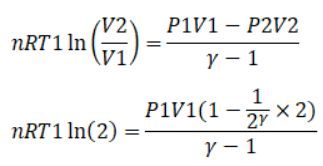For ideal gas equation, PV= nRT

ln(2) = [1- (1/2γ) x 2]/(γ-1)

=> ln 2(γ – 1) = 1 – 21-γ

Question 25: 1 litre of an ideal gas (γ = 1.5) at 300 K is suddenly compressed to half its original volume.

(a) Find the ratio of the final pressure to the initial pressure.

(b) If the original pressure is 100 kPa, find the work done by the gas in the process.

(c) What is the change in internal energy?

(d) What is the final temperature?

(e) The gas is now cooled to 300 K keeping its pressure constant.

Calculate the work done during the process.

(f) The gas is now expanded isothermally to achieve its original volume of 1 litre. Calculate the work done by the gas.

(g) Calculate the total work done in the cycle.

Solution:

Given : γ = 1.5 , T = 300 K and V2 = L/2

(a) In adiabatic process since volume is changed suddenly,

P2/P1 = (V1/V2)γ

=> P2/P1 = (2)1.5

(b)

Given: P1 = 100 KPa = 105 Pa, P2 = 21.5(105) KPa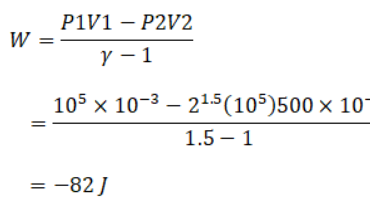(c) Since it is an adiabatic process, dQ = 0

By first law of thermodynamics, dQ = dU + dW

=> dU = -dW = 82 J

(d) In this case, for an adiabatic process, TV(γ-1) = constant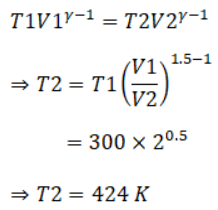(e) Work done in an isobaric process, here the pressure is kept constant.

W = P dV = nRdT

Here, n = PV/RT = [105 x 10-3]/[Rx300] = 1/3R

=> W = nRdT = (1/3R) x R x (300-424)

= -41.4 J

(f) In this case, process is isothermal.

Work done = nR dT ln(V2/V1) = (1/3R) x R x ln (2) = 103 J

(g) Total Work done in the cycle = -82 – 41.4 + 103 = -20.4 J